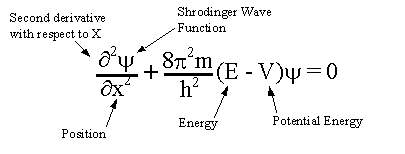# Schrodinger equation in 1 dimension Question details in the image?

## In this image E = V + KE KE = $\frac{1}{2} m {v}^{2}$ can you prove from this $\Delta x \Delta p \ge \frac{h}{4 \pi}$I try to solve this but instead end up proving that probablity of finding electron in all space is 1 p^2 * ψ^2 = p^2 This is the Schrodinger equation in 1 dimension I'm trying to prove this from various forms of the Schrodinger equation

Feb 3, 2018

Starting from

DeltaxDeltap_x >= ℏ"/"2

isn't actually enlightening. In fact it adds hours of pain and suffering because you have to derive it from scratch to find the form

$\Delta x \Delta {p}_{x} \ge \frac{1}{2} | i \left\langle\psi | \left[\hat{x} \text{,} {\hat{p}}_{x}\right] | \psi\right\rangle |$

where [hatx, hatp_x] = hatxhatp_x - hatp_xhatx = iℏ.

That would have shown that for normalized wave functions,

DeltaxDeltap_x >= 1/2 |i cdot i ℏ |

>= ℏ/2 sqrt(i^"*"i)

>= ℏ/2

Just know that the operators are:

$\hat{x} = x$

hatp_x = -iℏd/dx

which satisfy [hatx, hatp_x] = hatxhatp_x - hatp_xhatx = iℏ.

This is consistent with any Schrodinger equation in one Cartesian dimension, and is easy to prove. In fact, I have done it several times, and here is one of those times.

https://socratic.org/questions/in-heisenberg-s-uncertainty-principle-is-the-uncertainty-in-the-position-a-2-dim?source=search

Here is an easy proof to go from here...

We know:

• $p = m v$
• $K = \frac{1}{2} m {v}^{2}$

Thus,

hatK = hatp^2/(2m) = (-iℏ)^2 /(2m) del^2/(delx^2) = -ℏ^2/(2m) (del^2)/(delx^2)

So for any Schrodinger equation in one Cartesian dimension...

$\hat{H} \psi = E \psi$

$= \left(\hat{K} + \hat{V}\right) \psi$

= (-ℏ^2/(2m) (del^2)/(delx^2) + hatV)psi

Thus,

-ℏ^2/(2m) (del^2psi)/(delx^2) + hatVpsi = Epsi

(del^2psi)/(delx^2) - (2m)/(ℏ^2)[hatV-E]psi = 0

(del^2psi)/(delx^2) + (2m)/(ℏ^2)[E-hatV]psi = 0

$\textcolor{b l u e}{\frac{{\partial}^{2} \psi}{\partial {x}^{2}} + \frac{8 {\pi}^{2} m}{{h}^{2}} \left[E - \hat{V}\right] \psi = 0}$

This of course does NOT tell us what the potential is. That's your responsibility to tell us.